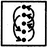# Non Verbal Reasoning - Series

### Exercise :: Series - Section 1

Each of the following questions consists of five figures marked A, B, C, D and E called the Problem Figures followed by five other figures marked 1, 2, 3, 4 and 5 called the Answer Figures. Select a figure from amongst the Answer Figures which will continue the same series as established by the five Problem Figures.

41.

Select a figure from amongst the Answer Figures which will continue the same series as established by the five Problem Figures.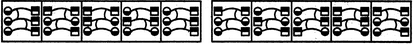(A)     (B)     (C)     (D)     (E)       (1)     (2)     (3)     (4)     (5)

 A. 1 B. 2 C. 3 D. 4 E. 5

Explanation:

The elements interchange positions in the orders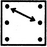and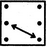alternately.

42.

Select a figure from amongst the Answer Figures which will continue the same series as established by the five Problem Figures.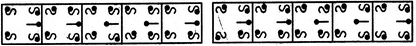(A)     (B)     (C)     (D)     (E)       (1)     (2)     (3)     (4)     (5)

 A. 1 B. 2 C. 3 D. 4 E. 5

Explanation:

The upper-left element gets laterally inverted in first, third, fifth. .... steps; the upper-right element gets rotated through 180o is first, fourth, seventh,.... steps; the lower-left element gets laterally inverted in second, fourth, sixth, ... steps; the lower-right element gets rotated through 180o in third, sixth,... steps and the pin at the middle-right position gets laterally inverted in every second step.

43.

Select a figure from amongst the Answer Figures which will continue the same series as established by the five Problem Figures.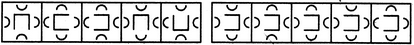(A)     (B)     (C)     (D)     (E)       (1)     (2)     (3)     (4)     (5)

 A. 1 B. 2 C. 3 D. 4 E. 5

Explanation:

Three and two arcs are inverted alternately. The central element rotates 90oACW and 180o alternately.

44.

Select a figure from amongst the Answer Figures which will continue the same series as established by the five Problem Figures.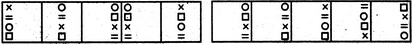(A)     (B)     (C)     (D)     (E)       (1)     (2)     (3)     (4)     (5)

 A. 1 B. 2 C. 3 D. 4 E. 5

Explanation:

All the elements together move one space to the right in each step and once they reach the rightmost position, then in the next step, they move to the leftmost position. Also, in the first step, the first (uppermost) and the third elements interchange positions; in the second step, the second and the fourth elements interchange positions and in the third step, none of the elements interchange positions. These three steps are repeated to continue the series.

45.

Select a figure from amongst the Answer Figures which will continue the same series as established by the five Problem Figures.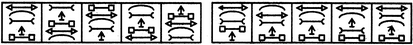(A)     (B)     (C)     (D)     (E)       (1)     (2)     (3)     (4)     (5)

 A. 1 B. 2 C. 3 D. 4 E. 5

In each step, the elements move in the order